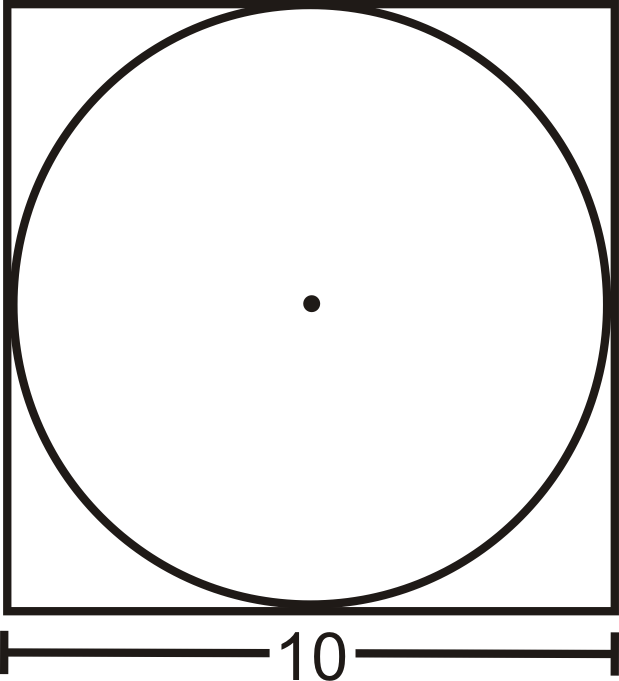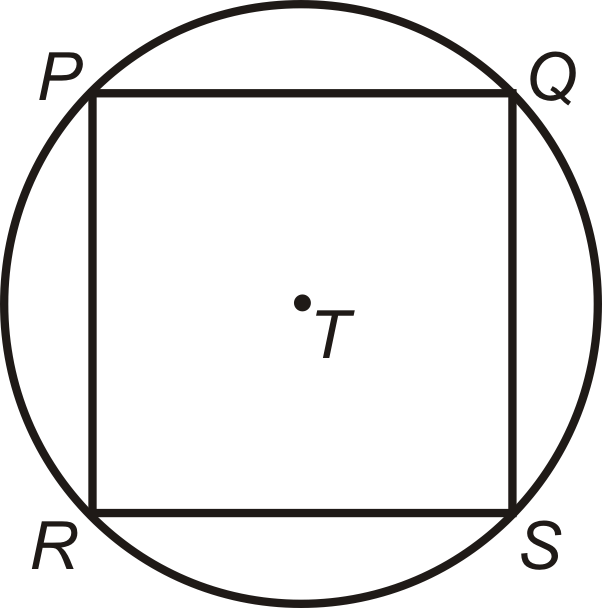# 6.4: Circumference

•• Contributed by CK12
• CK12

Find the circumference of a circle using the formulas: $$C = \pi d$$; $$C = 2\pi r$$.

Circumference is the distance around a circle. The circumference can also be called the perimeter of a circle. However, we use the term circumference for circles because they are round.

Circumference Formula: $$C=\pi d$$ where the diameter $$d=2r$$, or twice the radius. So $$C=2\pi r$$ as well.Figure $$\PageIndex{1}$$

$$\pi$$, or pi is the ratio of the circumference of a circle to its diameter. It is approximately equal to 3.14159265358979323846...

You should have a \pi button on your calculator. If you don't, you can use 3.14 as an approximation for $$\pi$$. You can also leave your answers in terms of $$\pi$$ for many problems.

What if you were given the radius or diameter of a circle? How could you find the distance around that circle?

Example $$\PageIndex{1}$$

Find the circumference of a circle with a radius of 7 cm.

Solution

Plug the radius into the formula.

$$C=2\pi (7)=14\pi \approx 44\text{ cm }$$

Example $$\PageIndex{2}$$

The circumference of a circle is $$64\pi$$ units. Find the diameter.

Solution

Again, you can plug in what you know into the circumference formula and solve for $$d$$.

\begin{aligned} 64\pi =\pi d \\ 64\text{ units }=d\end{aligned}

Example $$\PageIndex{3}$$

A circle is inscribed in a square with 10 in. sides. What is the circumference of the circle? Leave your answer in terms of $$\pi$$.Figure $$\PageIndex{2}$$

Solution

From the picture, we can see that the diameter of the circle is equal to the length of a side. $$C=10\pi \text{ in. }$$

Example $$\PageIndex{4}$$

Find the perimeter of the square in Example 3. Is it more or less than the circumference of the circle? Why?

Solution

The perimeter is $$P=4(10)=40\text{ in. }$$ In order to compare the perimeter with the circumference we should change the circumference into a decimal.

$$C=10\pi \approx 31.42 \text{ in. }$$ This is less than the perimeter of the square, which makes sense because the circle is inside the square.

Example $$\PageIndex{5}$$

The tires on a compact car are 18 inches in diameter. How far does the car travel after the tires turn once? How far does the car travel after 2500 rotations of the tires?Figure $$\PageIndex{3}$$

Solution

One turn of the tire is the circumference. This would be $$C=18\pi \approx 56.55\text{ in. }$$ 2500 rotations would be $$2500\cdot 56.55$$ in approx 141,375 in, 11,781 ft, or 2.23 miles.

## Review

Fill in the following table. Leave all answers in terms of $$\pi$$.

1. 15
2. 4
3. 6
4. $$84\pi$$
5. 9
6. $$25\pi$$
7. $$2\pi$$
8. 36
1. Find the circumference of a circle with $$d=\dfrac{20}{\pi} \text{ cm }$$.

Square $$PQSR$$ is inscribed in $$\bigodot T$$. $$RS=8\sqrt{2}$$.Figure $$\PageIndex{4}$$
1. Find the length of the diameter of $$\bigodot T$$.
2. How does the diameter relate to $$PQSR$$?
3. Find the perimeter of $$PQSR$$.
4. Find the circumference of $$\bigodot T$$.

For questions 14-17, a truck has tires with a 26 in diameter.

1. How far does the truck travel every time a tire turns exactly once? What is this the same as?
2. How many times will the tire turn after the truck travels 1 mile? (1 mile = 5280 feet)
3. The truck has travelled 4072 tire rotations. How many miles is this?
4. The average recommendation for the life of a tire is 30,000 miles. How many rotations is this?

## Vocabulary

Term Definition
chord A line segment whose endpoints are on a circle.
circle The set of all points that are the same distance away from a specific point, called the center.
Circumference The circumference of a circle is the measure of the distance around the outside edge of a circle.
diameter A chord that passes through the center of the circle. The length of a diameter is two times the length of a radius.
pi (or $$\pi$$ ) The ratio of the circumference of a circle to its diameter.
Radius The radius of a circle is the distance from the center of the circle to the edge of the circle.
Congruent Congruent figures are identical in size, shape and measure.
Regular Polygon A regular polygon is a polygon with all sides the same length and all angles the same measure.

Interactive Element

Video: Circumference of a Circle

Activities: Circumference Discussion Questions

study Aids: Circumference and Arc Length Study Guide

Practice: Circumference

Real World: A Roundabout Way Home